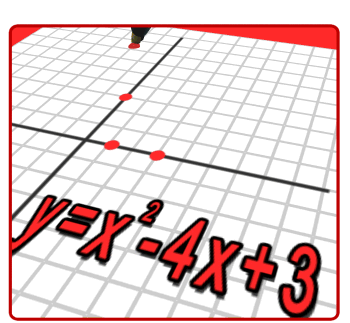• Welcome to Advanced Algebra IThis year we will be using the Algebra I book by Prentice Hall.  There are many videos and practice quizzes and tests that are available on the
Prentice Hall website

Here are the Chapters and topics we will be studying:

Chapter 1: Variables, Function Patterns, and Graphs

Chapter 2: Rational Numbers

Chapter 3: Solving Equations

Chapter 4: Solving Inequalities

Chapter 5: Graphs and Functions

Chapter 6: Linear Equations and Their Graphs

Chapter 7: Systems of Equations and Inequalities

Chapter 8: Exponents and Exponential Functions

Chapter 9: Polynomials and Factoring

Chapter 10: Quadratic Equations and Functions

Chapter 11: Radical Expressions and Equations

Chapter 12: Rational Expressions and Functions

*** RETESTS will be offered for End of Chapter tests. See the Retest policy